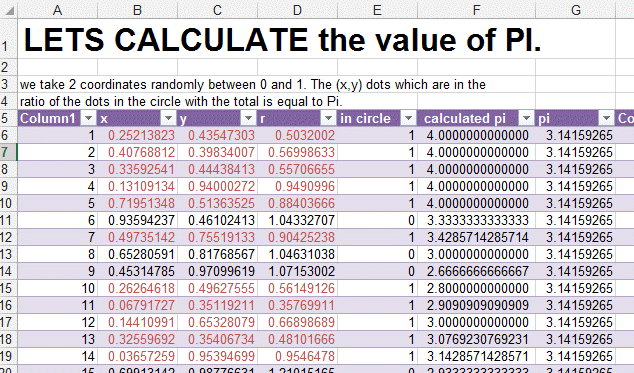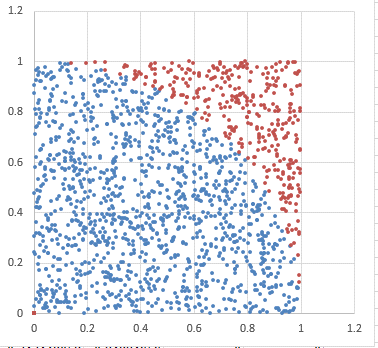## Example: Calculate Pi (3.1415926) in Microsoft Excel

You are wondering what is Pi and how it is calculated. Find out here.

Pi is the ratio of a circle and the square that surrounds this circle.

The area of a square is

Side x Side = Area

The Area of a circle is

PI x r^2

As the radius is equal to half the Side of the square. The area of the circle can be rewritten to

1/4 x Pi x Side^2

So the ration of the circle to the square is Pi/4.

Lets try this with Excel.

Beware the sheet has 1600 lines so it is quite big.and this is the GraphYou can see in blue the items in the circle and in red the items outside.

The ratio of Blue / (Blue+Red) = Pi/4

Have fun.

Here the value of Pi to a few hundreds digits....of course this example will not allow you this but one thing it will let you know

is if your random (RAND) number generator is of good quality. If it is, the value of PI should be approached quite fast. If not, then

the RAND generator cannot be trusted fully.

3.14159265358979323846264338327950288419716939937
510582097494459230781640628620899862803482534211
706798214808651328230664709384460955058223172535
940812848111745028410270193852110555964462294895
493038196442881097566593344612847564823378678316
527120190914564856692346034861045432664821339360
726024914127372458700660631558817488152092096282
925409171536436789259036001133053054882046652138
414695194151160943305727036575959195309218611738
1932611793105118548074462379962749567351885752...